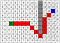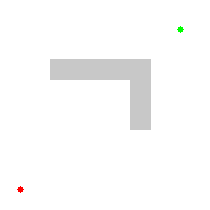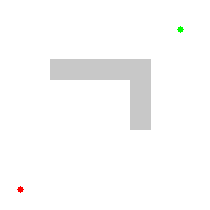# Easy A* (star) Pathfinding

Today we’ll being going over the A* pathfinding algorithm, how it works, and its implementation in pseudocode and real code with Python 🐍.

Looking for just pseudocode or source code? Scroll down!

If you’re a game developer, you might have always wanted to implement A* as character (or enemy) pathfinding. I know a couple of years ago I did, but with my level of programming at the time I had issues with the current articles out there. I wanted to write this as an easy introduction with a clear source code example to A* pathfinding so anyone can easily pick it up and use it in their game.

# F = G + H

• F is the total cost of the node.
• G is the distance between the current node and the start node.
• H is the heuristic — estimated distance from the current node to the end node.

Let’s take a look at a quick graphic to help illustrate this.wow such numbers, very color

Awesome! Let’s say `node(0)` is our starting position and `node(19)` is our end position. Let’s also say that our current node is at the the red square `node(4)`.

## G

G is the distance between the current node and the start node.

If we count back we can see that `node(4)` is 4 spaces away from our start node.

We can also say that G is 1 more than our parent node (`node(3)`). So in this case for `node(4)`, `currentNode.g = 4`.

## H

H is the heuristic — estimated distance from the current node to the end node.

So let’s calculate the distance. If we take a look we’ll see that if we go over 7 spaces and up 3 spaces, we’ve reached our end node (`node(19)`).

Let’s apply the Pythagorean Theorem! `a² + b² = c²`. After we’ve applied this, we’ll see that `currentNode.h = 7² + 3²`. Or `currentNode.h = 58`.

But don’t we have to apply the square root to 58? Nope! We can skip that calculation on every node and still get the same output. Clever!

With a heuristic, we need to make sure that we can actually calculate it. It’s also very important that the heuristic is always an underestimation of the total path, as an overestimation will lead to A* searching for through nodes that may not be the ‘best’ in terms of `f` value.

## F

F is the total cost of the node.

So let’s add up h and g to get the total cost of our node. `currentNode.f = currentNode.g + currentNode.h`. Or `currentNode.f = 4+ 58`. Or `currentNode.f = 62`.

# Time to use `f` = g + h

With this new `f` value, we can look at all our nodes and say, “Hey, is this the best node I can pick to move forward with right now?”. Rather than running through every node, we can pick the ones that have the best chance of getting us to our goal.

Here’s a graphic to illustrate. On top, we have Dijkstra’s Algorithm, which searches without this `f` value, and below we have A* which does use this `f` value.Dijkstra’s Algorithm (Wikipedia)A* Algorithm (Wikipedia)

Dijkstra’s Algorithm

So taking a look at Dijkstra’s algorithm, we see that it just keeps searching. It has no idea which node is ‘best’, so it calculates paths for them all.

A* Algorithm

With A*,we see that once we get past the obstacle, the algorithm prioritizes the node with the lowest `f` and the ‘best’ chance of reaching the end.

# A⭐️ Method Steps — from Patrick Lester

1. Add the starting square (or node) to the open list.

2. Repeat the following:

A) Look for the lowest F cost square on the open list. We refer to this as the current square.

B). Switch it to the closed list.

C) For each of the 8 squares adjacent to this current square …

• If it is not walkable or if it is on the closed list, ignore it. Otherwise do the following.
• If it isn’t on the open list, add it to the open list. Make the current square the parent of this square. Record the F, G, and H costs of the square.
• If it is on the open list already, check to see if this path to that square is better, using G cost as the measure. A lower G cost means that this is a better path. If so, change the parent of the square to the current square, and recalculate the G and F scores of the square. If you are keeping your open list sorted by F score, you may need to resort the list to account for the change.

D) Stop when you:

• Add the target square to the closed list, in which case the path has been found, or
• Fail to find the target square, and the open list is empty. In this case, there is no path.

3. Save the path. Working backwards from the target square, go from each square to its parent square until you reach the starting square. That is your path.

# Pseudocode

`// A* (star) Pathfinding// Initialize both open and closed listlet the openList equal empty list of nodeslet the closedList equal empty list of nodes// Add the start nodeput the startNode on the openList (leave it's f at zero)// Loop until you find the endwhile the openList is not empty    // Get the current node    let the currentNode equal the node with the least f value    remove the currentNode from the openList    add the currentNode to the closedList    // Found the goal    if currentNode is the goal        Congratz! You've found the end! Backtrack to get path    // Generate children    let the children of the currentNode equal the adjacent nodes        for each child in the children        // Child is on the closedList        if child is in the closedList            continue to beginning of for loop        // Create the f, g, and h values        child.g = currentNode.g + distance between child and current        child.h = distance from child to end        child.f = child.g + child.h        // Child is already in openList        if child.position is in the openList's nodes positions            if the child.g is higher than the openList node's g                continue to beginning of for loop        // Add the child to the openList        add the child to the openList`

Software Engineer at Facebook

## More from Nicholas Swift

Software Engineer at Facebook